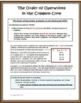# Order of Operations - A New Approach to Using and Understanding PEMDASSubject
Resource Type
Common Core Standards
Product Rating
File Type

PDF (Acrobat) Document File

1 MB|12 pages
Share
Product Description

Included in this ten page math resource is a lesson plan outline for introducing PEMDAS, and an easy to understand PEMDAS chart with an explanation of the Order of Operations for the student as well as practice problems. Unlike most PEMDAS charts which are aligned vertically, the one in this handout is designed so that the student will be reminded to work left to right when only addition and subtraction or multiplication and division remain.

Also contained is an explanation of how to properly use PEMDAS which may be copied on the back of the student chart. That way the student will have the chart (a visual) as well as the directions on how to use it. Ten practice problems plus one analysis question based on Bloom’s Taxonomy (essay) is part of the resource. All answers are included.

It is very important to introduce the Order of Operations early even if it is just using the operations of multiplication and division. Learning PEMDAS is implied in the third grade Common Core Standards (CCSS 3.OA.8). In the later grades, PEMDAS is reviewed and practiced often, but a correct introduction before fifth grade is needed to aid in the understanding of these rules. This resource is directly aligned with the fifth grade Common Core Standard 5.OA.1.

You might check out this game which requires the students to write three digit equations and use the Order of Operations.

Contact – A Math Game to Practice Math Facts and the Order of Operations

These resources might also be helpful:

Simple Math Formulas: Using a Triangular Graphic Organizer

Algebraic Terms and Fractions - Finding the Greatest Common Factor and Least Common Multiple Using a Venn Diagram

Total Pages
12 pages